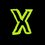# Annoying algebra in today's pre-RMO !

Hello !

If

$\sin^{-1} x + \sin^{-1} y + \sin^{-1} z = \dfrac{3 \pi}{2}$

Then find the value of :

$x^9 + y^9 + z^9 + \dfrac{1}{x^9y^9z^9}$

Well , I had got the following result from this : ( Might be wrong )

$x + y + z = -1$Note by Priyansh Sangule
6 years, 9 months ago

This discussion board is a place to discuss our Daily Challenges and the math and science related to those challenges. Explanations are more than just a solution — they should explain the steps and thinking strategies that you used to obtain the solution. Comments should further the discussion of math and science.

When posting on Brilliant:

• Use the emojis to react to an explanation, whether you're congratulating a job well done , or just really confused .
• Ask specific questions about the challenge or the steps in somebody's explanation. Well-posed questions can add a lot to the discussion, but posting "I don't understand!" doesn't help anyone.
• Try to contribute something new to the discussion, whether it is an extension, generalization or other idea related to the challenge.

MarkdownAppears as
*italics* or _italics_ italics
**bold** or __bold__ bold
- bulleted- list
• bulleted
• list
1. numbered2. list
1. numbered
2. list
Note: you must add a full line of space before and after lists for them to show up correctly
paragraph 1paragraph 2

paragraph 1

paragraph 2

[example link](https://brilliant.org)example link
> This is a quote
This is a quote
    # I indented these lines
# 4 spaces, and now they show
# up as a code block.

print "hello world"
# I indented these lines
# 4 spaces, and now they show
# up as a code block.

print "hello world"
MathAppears as
Remember to wrap math in $$ ... $$ or $ ... $ to ensure proper formatting.
2 \times 3 $2 \times 3$
2^{34} $2^{34}$
a_{i-1} $a_{i-1}$
\frac{2}{3} $\frac{2}{3}$
\sqrt{2} $\sqrt{2}$
\sum_{i=1}^3 $\sum_{i=1}^3$
\sin \theta $\sin \theta$
\boxed{123} $\boxed{123}$

Sort by:

The range of $\sin^{-1}$ is $[-\tfrac12\pi,\tfrac12\pi]$. Since $\sin^{-1}x+\sin^{-1}y+\sin^{-1}z = \tfrac32\pi$, we know the values of $\sin^{-1}x,\sin^{-1}y,\sin^{-1}z$ straight away (what three numbers between $-a$ and $a$ add to $3a$?). Thus we know $x,y,z$.

- 6 years, 9 months ago

Thank You !

- 6 years, 9 months ago

Very simple one! As, $\sin^{-1} x \to [\frac{- \pi}{2}, \frac{\pi}{2}]$

So, it leads us to the conclusion that $x=1, y=1, z=1$

Then, required value becomes $\boxed{4}$

- 6 years, 9 months ago

i thought same . quite easy.

- 6 years, 7 months ago

max value of the following equation is 3pi/2 and hence each angle should be pi/2. if angle is pi/2 then x=y=z=1 then 1 + 1 + 1 + 1 = 4. That's it

- 6 years, 9 months ago

What is Pre-RMO? Is the full paper available somewhere?

Thanks!

- 6 years, 9 months ago

Well this time , the government has allowed every state to set their own papers for pre-RMO . That is the qualification stage for RMO level 1 .

- 6 years, 9 months ago

In Telangana region,there is no pre-RMO....lucky for me I can directly write RMO paper without qualifying.... BTW....The RMO exam is not so easy as pre-RMO....I was dying to solve some of them...

- 6 years, 9 months ago

In Mumbai region there is Pre-RMO, as the no. of students taking part was much more. While the other regions in the country have RMO directly (including other-than-Mumbai Maharashtra part. We are going to give it on 1st December. Let's discuss some good problems thereafter...!!!

- 6 years, 9 months ago

Promo paper

- 2 years, 11 months ago

Priansh, can I get the copy of your PRMO paper??

- 6 years, 8 months ago

Well they didn't allow us to take it back home :/

- 6 years, 7 months ago